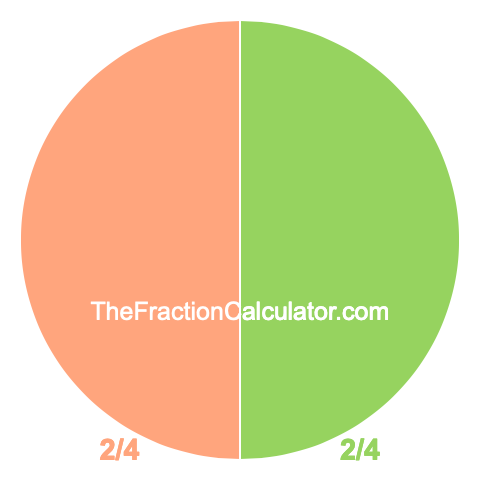Pie Chart of 2/4

To create a pie chart showing you how much 2/4 is, we first divided the pie (or circle) into 4 equal parts. Then we colored 2 of the 4 parts green. Here is our result:The pie chart of 2/4 not only gives you a visual of how much 2/4 of a whole is, but also shows you how much of the whole is not 2/4. To calculate what is not 2/4 of the pie chart, we simply subtracted 2/4 from one.

Pie Chart Fraction Maker
Get the pie chart for another fraction here. Make sure to submit a fraction where the numerator is smaller than the denominator.

/

Pie Chart of 2/5
Here is the next pie chart we have created for you.

Copyright  |   Privacy Policy  |   Disclaimer  |   Contact